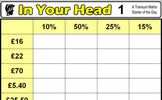# Fractions and percentages of amounts

### Term 2 starting in week 6 :: Estimated time: 1 weeks

• Find a fraction of a given amount
• Use a given fraction to find the whole and/or other fractions
• Find a percentage of a given amount using mental methods
• Find a percentage of a given amount using a calculator

For higher-attaining pupils:

• Solve problems with fractions greater than 1 and percentages greater than 100%

This page should remember your ticks from one visit to the next for a period of time. It does this by using Local Storage so the information is saved only on the computer you are working on right now.

## Lesson Starters

Here are some suggestions for whole-class, projectable resources which can be used at the beginnings of each lesson in this block.

### 1st Lesson#### Quick Percentages

Simple percentage questions appear on screen then fade every 8 seconds. This Starter is customisable.

### 2nd Lesson#### Odd Percent Out

A number of simple percentage calculations are given. The answers are in pairs. Which is the odd one out?

### 3rd LessonHere are the simple percentage calculations everyone should be able to do in their heads.

Some of the Starters above are to reinforce concepts learnt, others are to introduce new ideas while others are on unrelated topics designed for retrieval practice or and opportunity to develop problem-solving skills.

White Rose ResourcesEnd of block assessments provide a quick progress check at the end of each block of learning to make sure students have understood the content covered. This Scheme of Learning was produced by White Rose Maths and is used here with permission granted on 30th June 2021.For All: